# 57 and Cupid’s arrow is on its way!

57 is a composite number. 57 = 1 x 57 or 3 x 19. Factors of 57: 1, 3, 19, 57. Prime factorization: 57 = 3 x 19.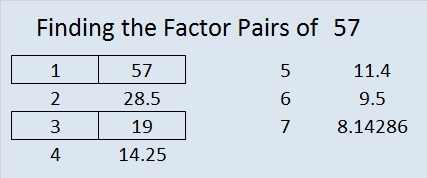57 is never a clue in the FIND THE FACTORS puzzles.

How do you feel about factoring?

If you will let Cupid hit you with this arrow, you might just fall in love with factors.

Factoring is very important in many levels of mathematics, so enjoying it can be a very good thing.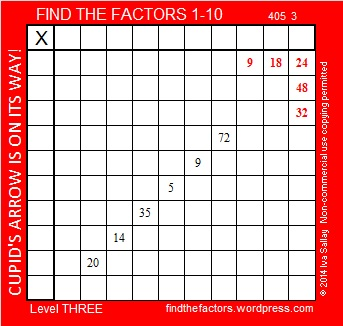I love factoring. I hope you will let Cupid’s arrow hit you so you will love factoring, too.

This week’s puzzles are available in an excel file here. If you have a spreadsheet program on your computer, you can access it. If you enable editing in excel, you can type your answers directly onto the puzzle, and you can also easily print the puzzles.

The factors for last week’s level 3 Puzzle:Here’s how last week’s puzzle was solved:# 54 and How Many Squares Are in This Puzzle?

### How Many Squares?

How many squares are in this puzzle? Finding that answer is too tedious for me to pursue. There is 1 square that is bigger than all the rest, 169 of the smallest size squares, and some different number between 1 and 169 for each size square in between. Also, I think the clues seem to form three little squares, and one of the clues, 16, happens to be a perfect square. It’s a trick question, and most people don’t like trick questions!

Solving the actual puzzle will actually be less work and more fun. The actual puzzle challenges you to write the numbers 1 through 12 in the top row and again in the first column so that the answers you write and the clues inside the puzzle work together as a multiplication table. Use logic to find the unique solution to the puzzle.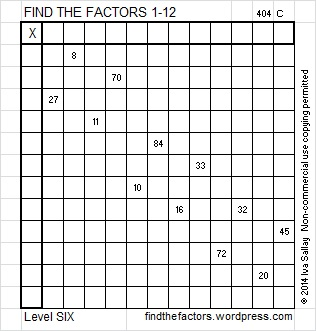This week’s puzzles are available in an excel file here. If you have a spreadsheet program on your computer, you can access it. If you enable editing in excel, you can type your answers directly onto the puzzle, and you can also easily print the puzzles.

### Factors of 54:

54 is a composite number. 54 = 1 x 54, 2 x 27, 3 x 18, or 6 x 9. Factors of 54: 1, 2, 3, 6, 9, 18, 27, 54. Prime factorization: 54 = 2 x 3 x 3 x 3, which can also be written 2 x 3³.Sometimes 54 is a clue in the FIND THE FACTORS puzzles. Even though it has other factors, the only multiplication fact the puzzle uses is 6 x 9 = 54.

### Sum-Difference Puzzle:

The number 6 has two factor pairs. One of those factor pairs adds up to 5, and the other one subtracts to 5. Can you put those factors in the correct places in the first puzzle?

The number 54 has four factor pairs. One of those factor pairs adds up to 15, and another one subtracts to 15. If you can identify those factor pairs, then you can solve the second puzzle.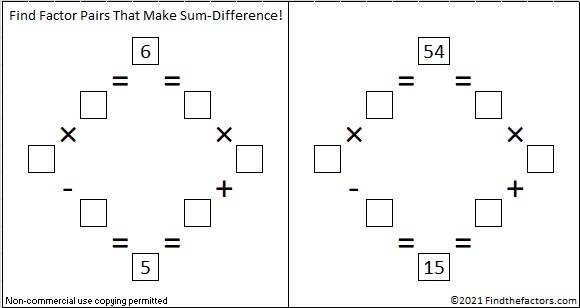The second puzzle is really just the first puzzle in disguise. Why would I say that?

###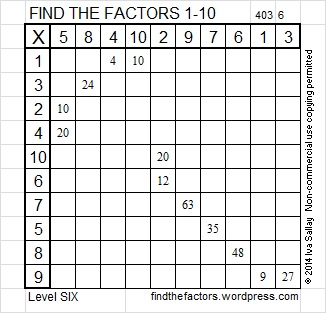Here is one way those factors can be found using logic.# 53 and Animated Gif FIND THE FACTORS Level 5

I’m a mother and a grandmother. My most recent grandson, Oliver, was born three days ago on Tuesday.Steve Wilhite, the creator of gif graphics format, said, “Choosy mothers choose GIF.” I think that can apply to grandmothers, too.

I made my very first animated gif! You can see the factors from last week’s level 5 puzzle appear one by one right before your eyes! I set the gif at the slowest possible setting, but it still goes fairly fast. The puzzle is solved from start to finish in about 15 seconds.Here is this week’s level 5 puzzle. To solve it, write the numbers from 1 to 12 in the top row and again in the first column so that the numbers you write are the factors of the given clues. There is only one solution.This week’s puzzles are available in an excel file here. If you have a spreadsheet program on your computer, you can access it. If you enable editing in excel, you can type your answers directly onto the puzzle, and you can also easily print the puzzles.

Here are the factors from last week’s level 5 puzzle:How were those factors found? Look at the animated gif above or click here to see the puzzle being solved, or you can look at the chart below for a slightly different way to solve it.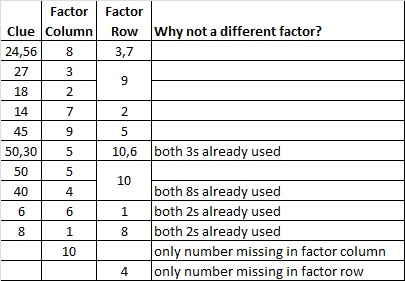• 53 is a prime number.
• Prime factorization: 53 is prime.
• The exponent of prime number 53 is 1. Adding 1 to that exponent we get (1 + 1) = 2. Therefore 53 has exactly 2 factors.
• Factors of 53: 1, 53
• Factor pairs: 53 = 1 x 53
• 53 has no square factors that allow its square root to be simplified. √53 ≈ 7.2801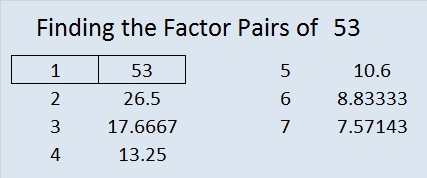How do we know that 53 is a prime number? If 53 were not a prime number, then it would be divisible by at least one prime number less than or equal to √53 ≈ 7.3. Since 53 cannot be divided evenly by 2, 3, 5, or 7, we know that 53 is a prime number.

# 50 and Multiples

Multiples and factors are related.

For example, 50 is a multiple of all these numbers: 1, 2, 5, 10, 25, and 50.

And 1, 2, 5, 10, 25, and 50 are all factors of 50.

50 is a composite number. 50 = 1 x 50, 2 x 25, or 5 x 10. Factors of 50: 1, 2, 5, 10, 25, 50. Prime factorization: 50 = 2 x 5 x 5, which can also be written 2 x 5².

The first few multiples of 50 are 50, 100, 150, 200, 250, 300, and so on…

If the difference between factors and multiples is confusing, this poster should help. Thanks to Resourceaholic for mentioning this great resource in one of her posts.Sometimes 50 is a clue in the FIND THE FACTORS puzzles. Even though it has other factors, we only use 50 = 5 x 10 to fill in the table.

Each of the clues inside this puzzle are MULTIPLES of a number from 1 to 12. Can you write every number from 1 to 12 in the top row as well as in the first column so that the clues are multiples of the numbers that you write?This week’s puzzles are available in an excel file here. If you have a spreadsheet program on your computer, you can access it. If you enable editing in excel, you can type your answers directly onto the puzzle, and you can also easily print the puzzles.

Here are the factors to last week’s level 4 puzzle: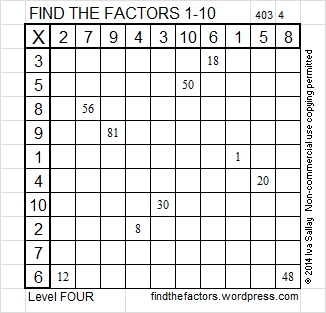The chart below shows one possible way to arrive logically at the solution.# 39 and Seriously Hooked on Factoring

39 is a composite number. 39 = 1 x 39 or 3 x 13. Factors of 39: 1, 3, 13, 39. Prime factorization: 39 = 3 x 13.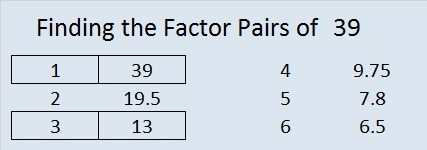39 is never a clue in the FIND THE FACTORS 1-10 or 1-12 puzzles.

Last Tuesday I published a hook-shaped level 2 puzzle and invited readers to get hooked on factoring. Today I present a level 6 hook-shaped puzzle. Admittedly, one would have to be seriously hooked on factoring to attempt and eventually complete the more difficult level 6 puzzle. I assure you that it can be solved using logic with absolutely no guessing and checking.This week’s puzzles are also available in an excel file here. If you have a spreadsheet program on your computer, you can access it. If you enable editing in excel, you can type your answers directly onto the puzzle, and you can also easily print the puzzles.

Here are the factors from last week’s level 6 snowball puzzle: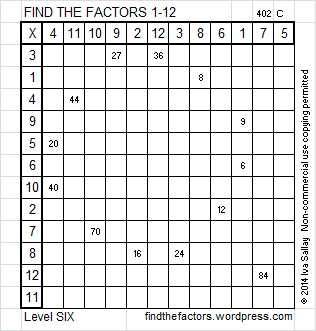Below is a table showing one possible way to solve the snowball puzzle using logic alone.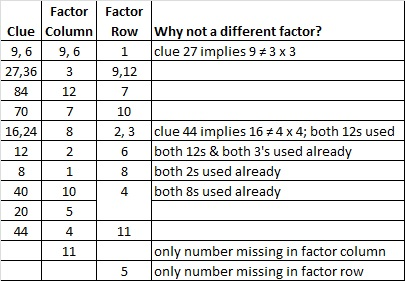Seriously, study the clues for this week’s puzzle and see if you can figure out how to solve it using logic alone. Guessing and checking will most likely only frustrate you. Good luck!

# 36 and The Six Word Challenge

36 is a composite number, and it is 6 squared. 36 = 1 x 36, 2 x 18, 3 x 12, 4 x 9, or 6 x 6. Factors of 36: 1, 2, 3, 4, 6, 9, 12, 18, 36. Prime factorization: 36 = 2 x 2 x 3 x 3, which can also be written 36 = 2² x 3².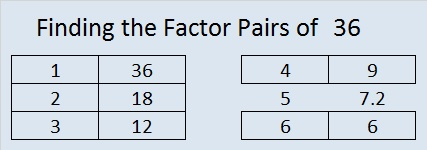Since √36 = 6, a whole number, 36 is a perfect square.

When 36 is a clue in the FIND THE FACTORS  1 – 10 puzzles, use either 4 x 9 or 6 x 6. When 36 is a clue in the FIND THE FACTORS 1 – 12 puzzles, use either 3 x 12, 4 x 9 or 6 x 6. Only one of those combinations will work for any particular puzzle.

I enjoy this multiplication rhyme: Six times six, magic tricks, abracadabra, thirty-six!

A fellow blogger issued a challenge for her readers to write a puzzling story or tell a story about a puzzle using exactly six words. I’m choosing to give puzzle directions today using these six words:

Without guessing, logically decide puzzle’s factors.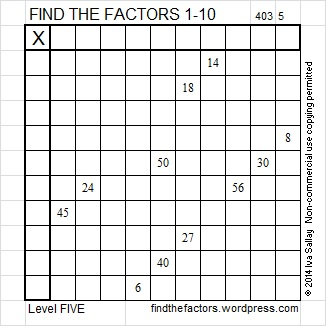It was a fun challenge. Her blog has a 6-word story written by Ernest Hemingway as well as a few written by other bloggers.

This week’s puzzles are also available in an excel file here. If you have a spreadsheet program on your computer, you can access it. If you enable editing in excel, you can type your answers directly onto the puzzle, and you can also easily print the puzzles.

Here are the factors for last week’s level 5 snowflake puzzle:There was more than one way to logically decide the snowflake puzzle’s factors. Some sets of clues needed to be avoided in the beginning: (18, 6) because both can be factored by 2, 3, and 6; (60, 40) because each can be factored by 5 and 10; and (16, 8) because both can be factored by 2, 4, and 8.

The column with 5 clues was a good place to start the puzzle after we narrowed down the common factor that would work for all the clues. The table below shows a way to find all the factors from 1 to 12 using logic without guessing and checking:Related article:

http://drieskewrites.wordpress.com/2014/01/22/six-word-story-challenge-puzzle/